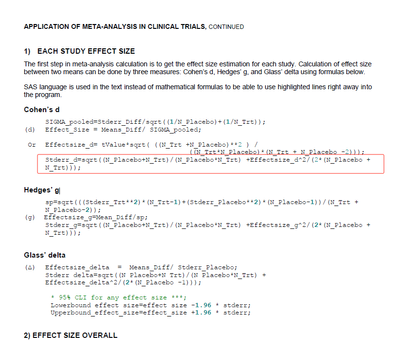## Calculating weight for site effect based on standard error

Hello, I am trying to explore the site effect in a clinical study where the site effect is significant. I have to find the weighted estimate using the proc mixed model "where the weight of each site is the inverse of the variance of each site estimate (which equals the inverse of the square of the standard error of each site estimate)."

Am I able to use the standard errors produced by the ls means statement to produce the  “standard error of each site estimate”?

9 REPLIES 9

## Re: Calculating weight for site effect based on standard error

@ashmork wrote:

Hello, I am trying to explore the site effect in a clinical study where the site effect is significant. I have to find the weighted estimate using the proc mixed model "where the weight of each site is the inverse of the variance of each site estimate (which equals the inverse of the square of the standard error of each site estimate)."

Am I able to use the standard errors produced by the ls means statement to produce the  “standard error of each site estimate”?

Is the reason you ask because you want to create a weighted regression? In that case, you can see a solution here: https://communities.sas.com/t5/SAS-Programming/Weighted-Least-Square-for-Simple-Linear-Regression/m-...

Or do you have some other reason for wanting this?

--
Paige Miller

## Re: Calculating weight for site effect based on standard error

Hello,
Thanks for your apply. Is this able to be done with a linear mixed model?

## Re: Calculating weight for site effect based on standard error

I don't understand. I don't see where you have answered my question.

--
Paige Miller

## Re: Calculating weight for site effect based on standard error

I might be able to do a regression model but my statistical plan specifically references a linear mixed model. So I wanted to know if this estimate, p-value, and CI can be done by a linear mixed model through proc mixed instead of proc reg?

## Re: Calculating weight for site effect based on standard error

You can use a WEIGHT in PROC MIXED, but I think it is computed the same as for linear regression or ANOVA. The weight is not based on the LSMEANs but on the actual data.

--
Paige MillerKsharp
Super User

## Re: Calculating weight for site effect based on standard error

Are you doing META analysis ?
And trying to get stderr of effect size estimation for each siteid  ?
By my side, I think your are right, you could use stderr generated by LSMEANS .

But @SteveDenham , @lvm @StatDave know more things.Ksharp
Super User

## Re: Calculating weight for site effect based on standard error

If you are doing META Analysis ,you could try this formula:## Re: Calculating weight for site effect based on standard error

It is not clear what you are trying to do here. It appears to me that you want to do a meta-analysis. That is, you have results from each site (means, SEs, ...), and now you want to combine to determine an overall mean and SE (effect size mean for the population of sites). If that is what you are doing, MIXED or GLIMMIX can certainly be used, where one overrides the residual with a weight term. There are tricks to the coding that are not obvious. But I don't know if that is what you are trying to do. It is possible that you simply want a hierarchical linear mixed model, but with separate residual for each site, all in one analysis (no meta-analysis). That also can be done, where you get a different residual for each site (sort of like using weights).

I can't give you code until you more fully describe what you are trying to do. Maybe with a toy example.SteveDenham

## Re: Calculating weight for site effect based on standard error

Hmm, that does depend on what your objective is, but I will take a swing at it without knowing what your data is (site means, SDs and Ns, or raw data).

The point I want to address is whether to treat site as a fixed effect (inference is only to the sites included) or a random effect (inference is to all possible sites). All of this assumes using PROC GLIMMIX, as I am fairly certain you are not looking at data with normally distributed residuals if it is primary endpoint clinical data. So, considering SITE as a fixed effect, here is code that will yield a variance estimate for each site:

``````proc glimmix data=your_data;
class site treatment;
model response = treatment/dist= <insert appropriate distribution here>;
random _residual_/group=site;
run;``````

For SITE as a random effect, you could try:

``````proc glimmix data=your_data;
class site treatment;
model response = treatment/dist= <insert appropriate distribution here>;
random intercept/subject=site solution;
run;``````

Using ODS output statements, you can get the variance estimates for each site from code like this. Weights can then be calculated as 1/variance, merged back against the original dataset, and the data refit using a WEIGHT statement. The Type 3 F test for this analysis reflects any differences in treatment effect after weighting by site.

I am not a clinical biostatistician, but the meta-analyses I have seen generally treat site as a fixed effect. You might want to talk with others about the choice.

SteveDenham

Discussion stats
• 9 replies
• 423 views
• 0 likes
• 5 in conversation